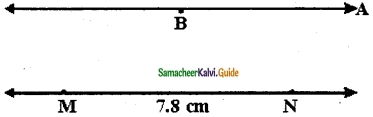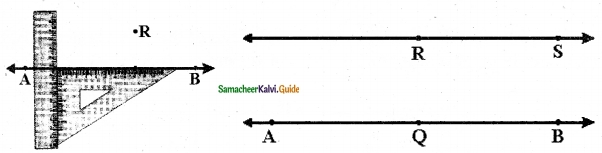Students can download Maths Chapter 4 Geometry Ex 4.2 Questions and Answers, Notes, Samacheer Kalvi 6th Maths Guide Pdf helps you to revise the complete Tamilnadu State Board New Syllabus, helps students complete homework assignments and to score high marks in board exams.

## Tamilnadu Samacheer Kalvi 6th Maths Solutions Term 2 Chapter 4 Geometry Ex 4.2

Question 1.
Draw a line segment AB = 7 cm and mark a point P on it. Draw a line perpendicular to the given line segment at P.
Solution:Step 1 : Draw a line AB = 7 cm and take a point P anywhere on the line.
Step 2 : Place the set square on the line in such a way that the vertex which forms right angle coincides with P and one arm of the right angle coincides with the line AB.
Step 3 : Draw a line PQ through P along the other arm of the right angle of the set square.
Step 4 : The line PQ is perpendicular to the line AB at P. That is, PQ ⊥ AB
∠APQ = ∠BPQ = 90°Question 2.
Draw a line segment LM = 6.5 cm and mark a point X not lying on it. Using a set square construct a line perpendicular to LM through X.
Solution:Step 1 : Draw a line LM = 6.5 cm and take a point X anywhere above the line LM.
Step 2 : Place one of the arms of the right angle of a set square along the line LM and the other arm of its right angle touches the point X.
Step 3 : Draw a line through the point X meeting LM at Y.
Step 4 : The line XY is perpendicular to the line LM at Y. That is, LM ⊥ XY.Question 3.
Find the distance between the given lines using a set square at two different points on each of the pairs of lines and check whether they are parallel.Solution:
They are parallelQuestion 4.
Draw a line segment measuring 7.8 cm. Mark a point B above it at a distance of 5 cm. Through B draw a line parallel to the given segment.
Solution:Step 1 : Draw a line. Mark two points M and N on the line such that MN = 7.8 cm. Mark a point B any where above the line.
Step 2 : Place the set square below B in such a way that one of the edges that form a right angle lies along MN Place the scale along the other edge of the set square.
Step 3 : Holding the scale firmly, Slide the set square along the edge of the scale until the other edge of the set square reaches the point B. Through B draw a line.
Step 4 : The line MN is parallel to AB. That is, MN || AB.Question 5.
Draw a line and mark a point R above it at a distance of 5.4 cm Through R draw a line parallel to the given line.
Solution:Step 1 : Using a scale draw a line AB and mark a point Q on the line.
Step 2 : Place the set square in such a way that the vertex of the right angle coincides with Q and one of the edges of right angle lies along AB. Mark the point R such that QR = 5.4 cm
Step 3 : Place the scale and the set square as shown in the figure.
Step 4 : Hold the scale firmly and slide the set square along the edge of the scale until the other edge touches the point R. Draw a line RS through R.
Step 5 : The line RS is parallel to AB. That is, RS || AB.